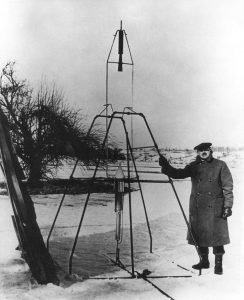# A simple Goddard problem

Goddard problemThis well-known problem (see for instance ,) models the ascent of a rocket through the atmosphere, and we restrict here ourselves to  vertical (monodimensional) trajectories.
The state variables are the altitude, speed and mass of the rocket during the flight, for a total dimension of 3. The rocket is subject to gravity, thrust and drag forces. The final time is free, and the objective is to reach a certain altitude with a minimal fuel consumption, ie a maximal final mass. All units are renormalized.$Latex formula$

The drag is$Latex formula$, with the volumic mass is$Latex formula$.
We use the parameters$Latex formula$.

The Hamiltonian is an affine function of the control, so singular arcs may occur. We consider here a path constraint limiting the value of the drag effect:$Latex formula$. We see that depending on the value of C, the control structure changes. In the unconstrained case, the optimal trajectory presents a singular arc with a non-maximal thrust. When C is set under the maximal value attained by the drag in the unconstrained case, a constrained arc appears. If C is small enough, the singular arc is completely replaced by the constrained arc.

Numerical simulations:
Discretization: Gauss 4th order with 1000 steps.
Depending on the value of C, the optimal control structure changes from Bang-Singular-Bang to Bang-Constrained-Bang.

References

 R.H. Goddard. A Method of Reaching Extreme Altitudes, volume 71(2) of Smithsonian Miscellaneous Collections. Smithsonian institution, City of Washington, 1919.

 H. Seywald and E.M. Cliff. Goddard problem in presence of a dynamic pressure limit. Journal of Guidance, Control, and Dynamics, 16(4):776–781, 1993.